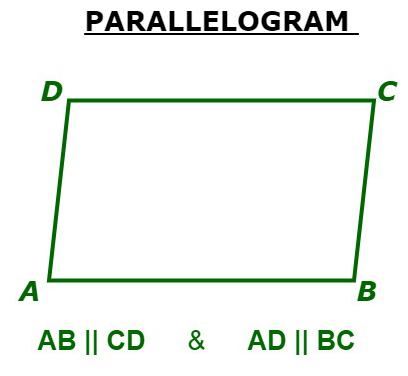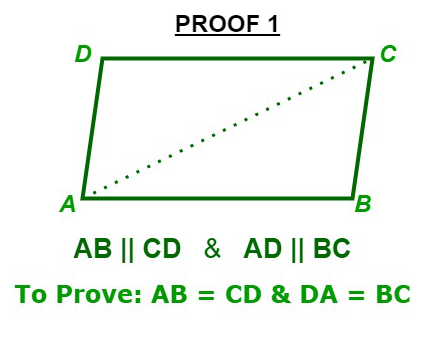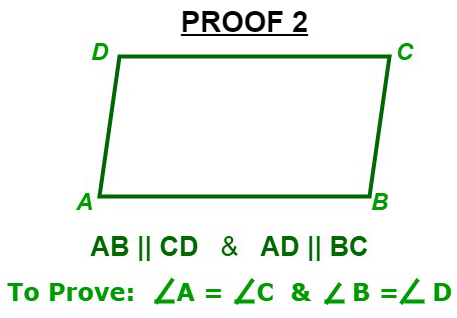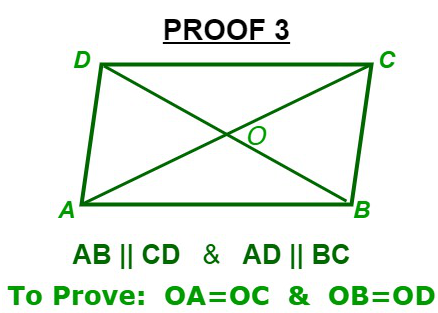Related Articles

# Properties of Parallelograms

• Last Updated : 19 Jan, 2021

A quadrilateral having both the pairs of opposite sides equal is a parallelogram. A parallelogram is a two-dimensional geometrical shape, whose sides are parallel to each other. Below are some simple facts about parallelogram:

1. Number of sides in Parallelogram = 4
2. Number of vertices in Parallelogram = 4
3. Area = Base x Height
4. Perimeter = 2 (Sum of adjacent sides length)
5. Type of polygon = Quadrilateral

Below is the representation of a parallelogram:## Proofs: Parallelograms

### Proof 1: Opposite sides of a parallelogram is equal.Given: ABCD is a parallelogram

To Prove: AB = CD & DA = BC

Firstly, Join AC

As given ABCD is a parallelogram. Therefore,

AB || DC  &  AD || BC

Now,  AD || BC and AC is intersecting A and C respectively.DAC =BCA                   …(i)                  [Alternate Interior Angles]

Now, AB || DC and AC is intersecting A and C respectively.BAC =DCA                 …(ii)                      [Alternate Interior Angles]

Now, InADC &CBADAC =BCA                    [ From (i) ]

AC = AC                                   [ Common Side ]DCA =BAC                 [ From (ii) ]

So, by ASA(Angle-Side-Angle) criterion of congurenceADCCBA

AB = CD & DA = BC [ Corresponding part of congurent triangles are equal ]

Hence Proved !

### Proof 2: Opposte angles of a parallelogram are equal.Given: ABCD is a parallelogram

To Prove:A =C  andB =D

As given ABCD is a parallelogram. Therefore,

AB || DC  &  AD || BC

Now, AB || DC and AD is Intersecting them at A and D respectively.A +D = 180…(i)             [ Sum of consecutive interior angles is 180]

Now, AD || BC and DC is Intersecting them at D and C respectively.D +C = 180…(ii)            [ Sum of consecutive interior angles is 180\degree]

From (i) and (ii) , we getA +D =D  +C

So,A =C

Similarly,B =DA =C andB =D

Hence Proved !

### Proof 3: Diagonals of a parallelogram bisect each other.Given: ABCD is a parallelogram

To Prove: OA = OC & OB = OD

As given ABCD is a parallelogram. Therefore,

AB || DC  &  AD || BC

Now, AB || DC and AC is intersecting A and C respectively.BAC =DCA                               [ Alternate Interior Angles are equal ]

So,BAO =DCO

Now,  AB || DC and BD is intersecting B and D respectively.ABD =CDB                               [ Alternate Interior Angles are equal ]

So,ABO =CDO

Now, inAOB &COD we have,BAO =DCO                               [ Opposite sides of a parallelogram are equal ]

AB = CDABO =CDO

So, by ASA(Angle-Side-Angle) congurence criterionAOBCOD

OA = OC and OB = OD

Hence Proved !

Attention reader! Don’t stop learning now. Participate in the Scholorship Test for First-Step-to-DSA Course for Class 9 to 12 students.

My Personal Notes arrow_drop_up